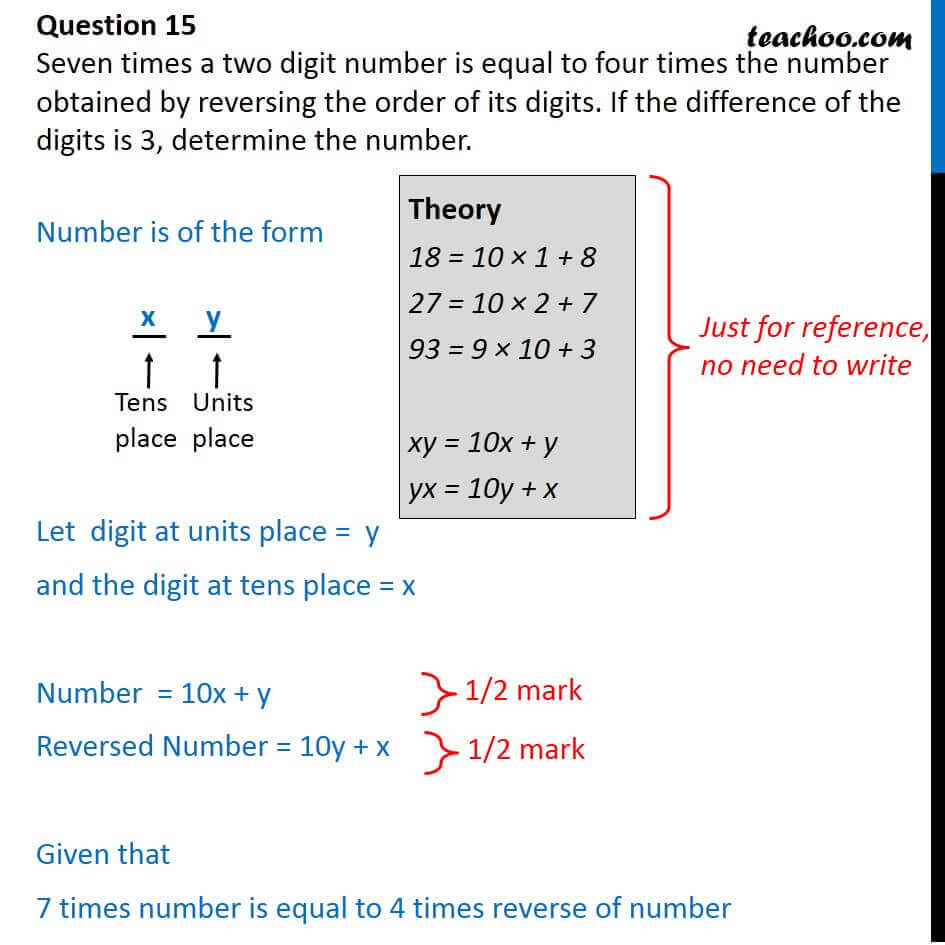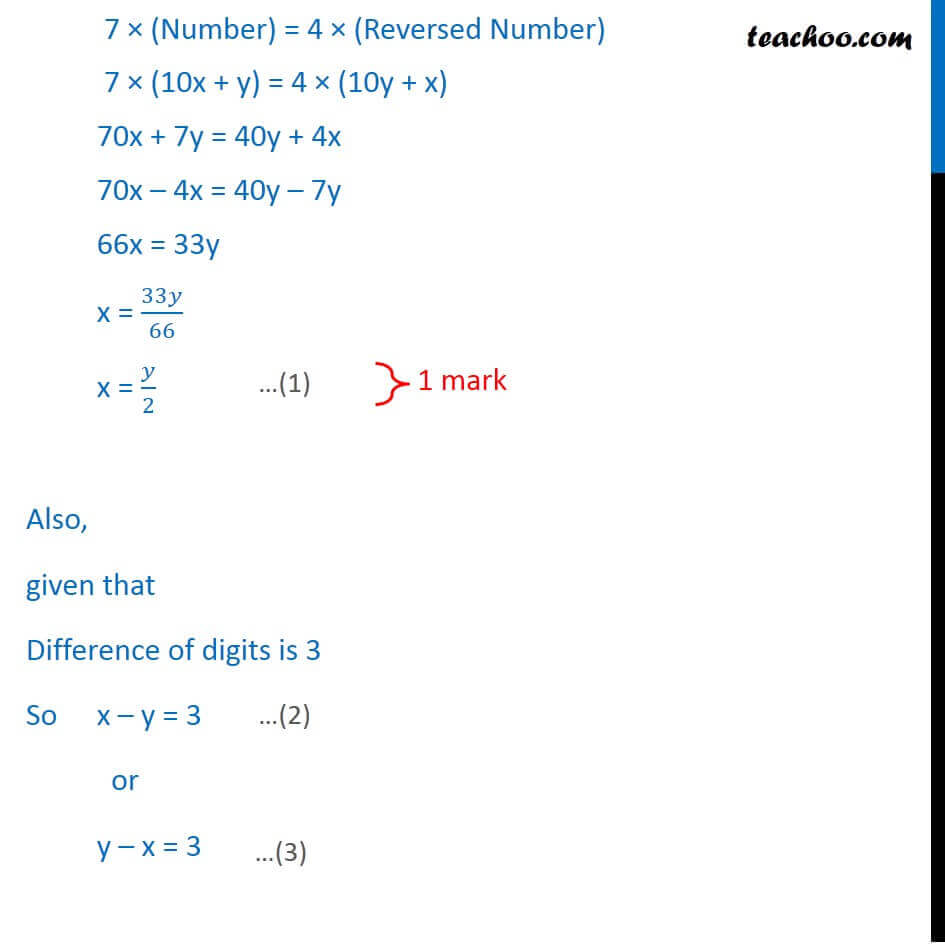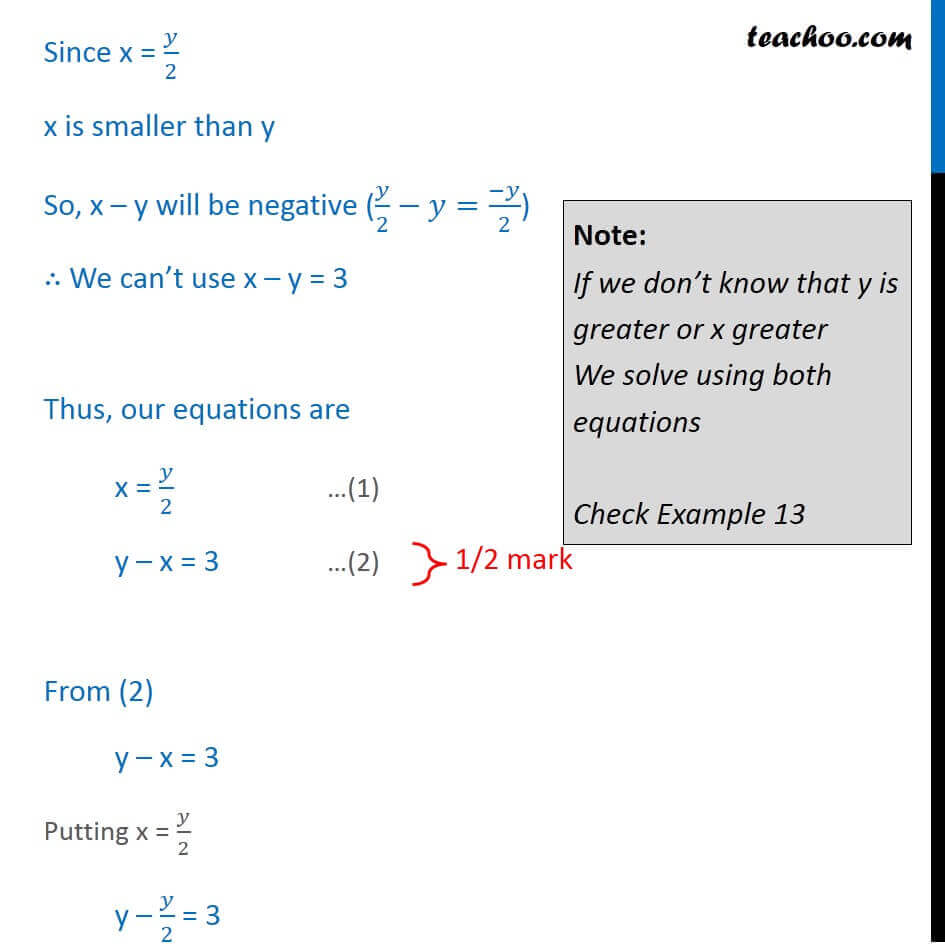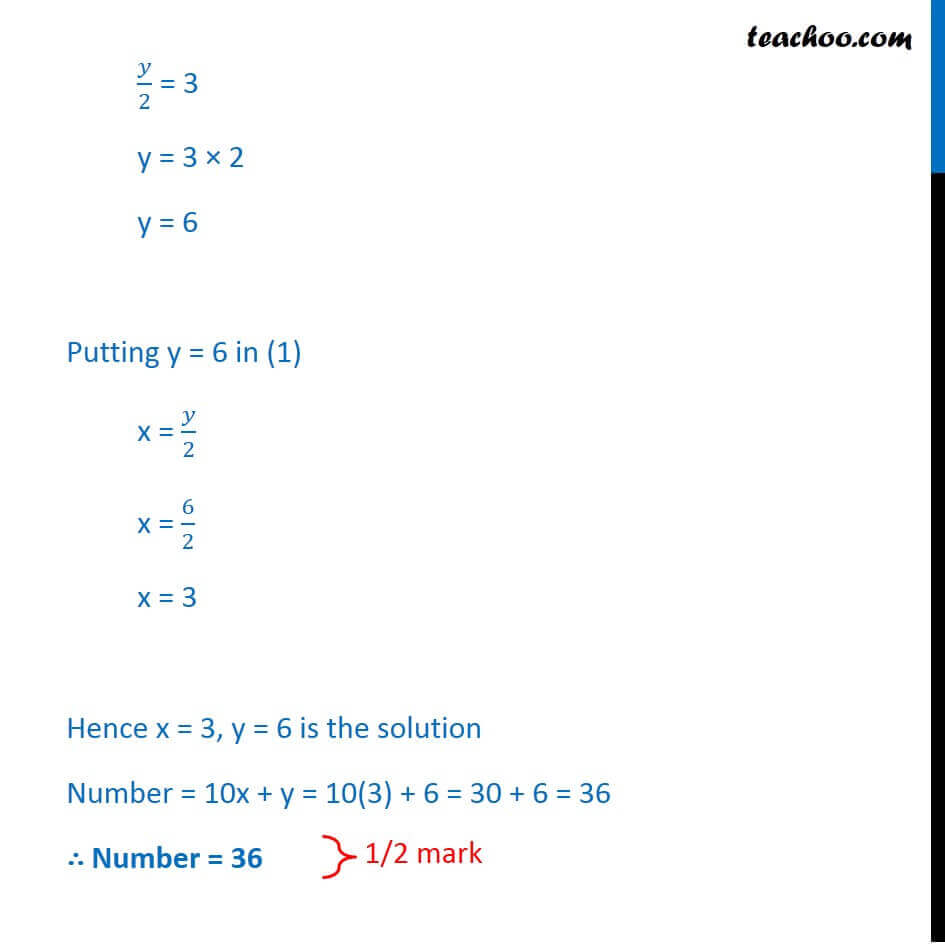CBSE Class 10 Sample Paper for 2018 Boards

Class 10
Solutions of Sample Papers for Class 10 Boards

### Seven times a two digit number is equal to four times the number obtained by reversing the order of its digits. If the difference of the digits is 3, determine the number.

This is a question of CBSE Sample Paper - Class 10 - 2017/18.Note:

If we don’t know that y is greater or x greater

We solve using both equationsLearn in your speed, with individual attention - Teachoo Maths 1-on-1 Class

### Transcript

Question 15 Seven times a two digit number is equal to four times the number obtained by reversing the order of its digits. If the difference of the digits is 3, determine the number. Number is of the form Let digit at units place = y and the digit at tens place = x Number = 10x + y Reversed Number = 10y + x Given that 7 times number is equal to 4 times reverse of number 7 (Number) = 4 (Reversed Number) 7 (10x + y) = 4 (10y + x) 70x + 7y = 40y + 4x 70x 4x = 40y 7y 66x = 33y x = 33 /66 x = /2 Also, given that Difference of digits is 3 So x y = 3 or y x = 3 Since x = /2 x is smaller than y So, x y will be negative ( /2 =( )/2) We can t use x y = 3 Thus, our equations are x = /2 (1) y x = 3 (2) From (2) y x = 3 Putting x = /2 y /2 = 3 /2 = 3 y = 3 2 y = 6 Putting y = 6 in (1) x = /2 x = 6/2 x = 3 Hence x = 3, y = 6 is the solution Number = 10x + y = 10(3) + 6 = 30 + 6 = 36 Number = 36